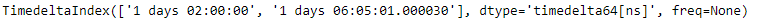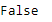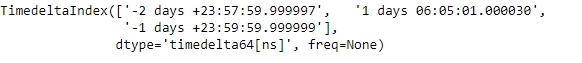Open in App
Not now

# Python | Pandas TimedeltaIndex.empty

• Last Updated : 29 Dec, 2018

Python is a great language for doing data analysis, primarily because of the fantastic ecosystem of data-centric python packages. Pandas is one of those packages and makes importing and analyzing data much easier.

Pandas` TimedeltaIndex.empty` attribute return a boolean value. It returns `False` if the TimedeltaIndex object on which it is applied is not empty.

Syntax: TimedeltaIndex.empty

Return : dtype

Example #1: Use `TimedeltaIndex.empty` attribute to check if the given TimedeltaIndex object is empty or not.

 `# importing pandas as pd``import` `pandas as pd`` ` `# Create the TimedeltaIndex object``tidx ``=` `pd.TimedeltaIndex(data ``=``[``'1 days 02:00:00'``, ``'1 days 06:05:01.000030'``])`` ` `# Print the TimedeltaIndex``print``(tidx)`

Output :Now we will check if the given TimedeltaIndex object is empty or not.

 `# check if tidx is empty or not``tidx.empty`

Output :As we can see in the output, the `TimedeltaIndex.empty` has returned `False` indicating that tidx object is not empty.

Example #2: Use `TimedeltaIndex.empty` attribute to check if the given TimedeltaIndex object is empty or not.

 `# importing pandas as pd``import` `pandas as pd`` ` `# Create the TimedeltaIndex object``tidx ``=` `pd.TimedeltaIndex(data ``=``[``'-1 days 2 min 3us'``, ``'1 days 06:05:01.000030'``,``                                                  ``'-1 days + 23:59:59.999999'``])`` ` `# Print the TimedeltaIndex``print``(tidx)`

Output :Now we will check if the given TimedeltaIndex object is empty or not.

 `# check if tidx is empty or not``tidx.empty`

Output :As we can see in the output, the `TimedeltaIndex.empty` has returned `False` indicating that tidx object is not empty.

My Personal Notes arrow_drop_up